## Statistical Tests using Python

By: Ram on Apr 04, 2021

## T- Test

T-Test checks if two samples are drawn from the same population and ist is validated comparing the means of the two samples.

H0: Two-Sample Means are Same (This is called the null hypothesis)

H1: Two-Sample Means are not the same (This is called an alternate hypothesis)

It is assumed that the error follows Student's T Distribution. The hypothesis can be evaluated based on:

• Comparing calculated T Statistics with critical values
• P-Value and Alpha Value comparison

Here is a guideline to accept the null hypothesis

• abs(t-statistic) <= critical value: Accept null hypothesis
• p > alpha: Accept the null hypothesis

Scenario: We have two segments of customers - Attrition and Non-Attrition. We want to check if Age distribution is different for these two segments.

If these two segments are similar based on Age (or they are representing the same population), the mean of these two samples will be the same.

H0: Customers who have attrited and non-attrited have the same means.

`from google.colab import drive`

`drive.mount('/content/drive')`

`import pandas as pd`

`import zipfile`

`# Create reference to zipped file/folder`

`mr = zipfile.ZipFile('/content/drive/MyDrive//Training/ML - Mar2021/Data/Predicting Churn for Bank Customers.zip')`

`def BiVarContPlot(df, depVar, feature):  `

`  import matplotlib.pyplot as plt`

`  import seaborn as sns`

`  colors = ['Green', 'Orange']`

`  df_1 =  df[df[depVar]==1]`

`  df_0 =  df[df[depVar]==0]`

`  fig, (ax1, ax2) = plt.subplots(1, 2, figsize = (12, 4))    `

`  sns.distplot(df_0[feature], bins = 15, color = colors, label = 'Not Attrited', hist_kws = dict(edgecolor = 'firebrick', linewidth = 1), ax = ax1, kde = False)`

`  sns.distplot(df_1[feature], bins = 15, color = colors, label = 'Attrited', hist_kws = dict(edgecolor = 'firebrick', linewidth = 1), ax = ax1, kde = False)`

`  ax1.set_title('{} distribution - Histogram'.format(feature))`

`  ax1.set_ylabel('Counts')`

`  ax1.legend()`

`  sns.boxplot(x = 'Exited', y = feature, data = df, palette = colors, ax = ax2)`

`  ax2.set_title('{} distribution - Box plot'.format(feature))`

`  ax2.set_xlabel('Status')`

`  ax2.set_xticklabels(['Non Attrited', 'Attrited'])`

#### Visualize Distribution: Age for Two Segments/Samples

`BiVarContPlot(attrition,'Exited','Age')`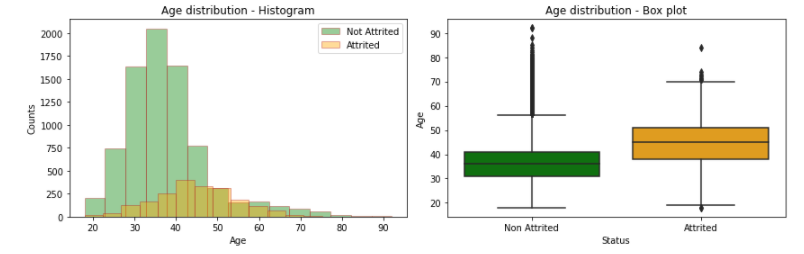#### T-Test

It is evident from the visualization the average age for these two segments/samples is different. But we want to validate if this difference is statistically significant.

`from scipy import stats`

`seg1 = attrition.Age[attrition.Exited==0]`

`seg2 = attrition.Age[attrition.Exited==1]`

`print('Mean for Non Attrition Segment:',seg1.mean())`

`print('Mean for Attrition Segment:',seg2.mean())`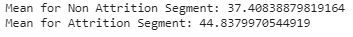#### T-Test using Python

`stats.ttest_ind(seg1, seg2)`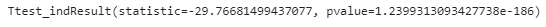Now, we need to make a conclusion based on the calculated T-Test Statistic and P-Value.

For the 5% significance level, the value of Alpha is 0.05.

Since P-Value is lower than Alpha (0.05), we reject the null hypothesis. So, these two samples are not coming from the same population. Or the age difference between these two segments is significant.

## Analysis of Variance (ANOVA)

Analysis of Variance is applied to compare means across 2 or more samples/groups using the variance of the samples/groups

In common applications of ANOVA, the dependent variable is continuous and the independent variable is a categorical (Nominal or Ordinal) variable. The mean of dependent variables is compared across categorical variable values

Typically, the Null hypothesis is that the means are the same across the groups. If the number of groups across which mean values are compared is 2, the result of ANOVA analysis is similar or the same as that of the t-test

ANOVA tests Ho: all group means are equal vs. Ha: at least one group’s mean is differentScenario: In the above data, we have Geographic features and takes 3 values - France, Spain, and Germany.

We want to understand if the Average Age of Customers across these 3 segments is statistically different

`# Prepare Data`

`data = [attrition['Age'][attrition['Geography'] == 'France'],`

`        attrition['Age'][attrition['Geography'] == 'Germany'],`

`        attrition['Age'][attrition['Geography'] == 'Spain']]`

`labels =['France','Germany','Spain']`

`# Plot `

`import matplotlib.pyplot as plt`

`fig = plt.figure(figsize= (10, 10))`

`ax = fig.add_subplot(111)`

`ax.set_title("Box Plot of Geography", fontsize= 20)`

`ax.boxplot(data,`

`           labels= labels,`

`           showmeans= True)`

`plt.xlabel("Geo")`

`plt.ylabel("Age")`

`plt.show()`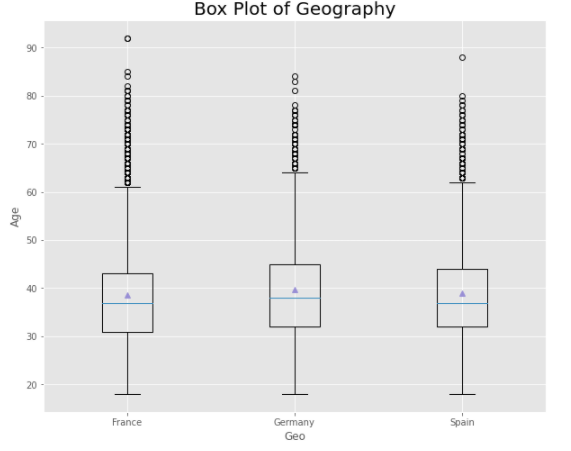#### ANOVA in Python

`# Creates the ANOVA table`

`import statsmodels.api as sm`

`from statsmodels.formula.api import ols`

`model = ols('Age ~ C(Geography)', attrition).fit()`

`model.summary()`

`res = sm.stats.anova_lm(model, typ= 2)`

`res`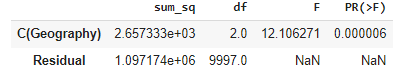### Steps in ANOVA

Step 1: Total Sum of Square

`# Overall Mean`

`overall_mean = attrition.Age.mean()`

`# Square of Variance`

`variance_square = (attrition.Age-overall_mean)**2`

`# Total Sum of Square `

`sst = variance_square.sum()`

#### Step 2: Among Group Sum of Square

`# Group 1`

`g1 = attrition.Age[attrition.Geography=='France']`

`avg_g1 = g1.mean()`

` `

`ssg =len(g1)*(avg_g1-overall_mean)**2 + len(g2)*(avg_g2-overall_mean)**2 + len(g3)*(avg_g3-overall_mean)**2`

`# Group 2`

`g2 = attrition.Age[attrition.Geography=='Spain']`

`avg_g2 = g2.mean()`

`# Group 3`

`g3 = attrition.Age[attrition.Geography=='Germany']`

`avg_g3 = g3.mean()`

#### Step 3: Error Sum of Square

Total Sum of Square (SST) = Sum of Square Among Group (SSG)+ Sum of Square for Error (SSE)

SST = SSG+SSE SSE = SST - SSG

`sse = sst-ssg`

#### Step 4: F Statistics and P-Value

Degree of Freedom (total): N-1

Degree of Freedom (groups): G-1

Degree of Freedom (Error): N-G

Mean of Sum of Square for Total (MST) = SST/N-1

Mean of Sum of Square Among Groups (MSG) = SSG/G-1

Mean of Sum of Square for Errors (MSE) = SSE/N-G

F = MSG/MSE

`n = len(attrition.Age)`

`g = 3`

`mst = sst/(n-1)`

`msg = ssg/(g-1)`

`mse = sse/(n-g)`` `

`f= msg/mse`

`f`

12.106270732486

#### Scenario for ANOVA Application

When a customer or individual takes up an insurance policy, it pays a premium and applies for insurance claims as per insurance policy details. The example is of Health Insurance and details include policy inception year (the year when a policy is taken or become effective) and claim amount. It is important for the business to understand how the average claim amount varies across policy inception years or geographical locations of the customers. Based on the initial ANOVA test results further analysis can be carried out to understand the drivers of claim amount and profiles of the customers acquired across the years.

## Chi-Square Test

Pearson's chi-squared test (χ2) / Chi-Square test is a statistical test applied to sets of categorical data to measure the association between different values of a variable (one variable) or between categories of different variables.

The Chi-Squared test is a statistical hypothesis test. It evaluates whether observed frequencies match expected frequencies for the categorical variables.

H0: Observed Frequency Matches Expected Frequencies (Null Hypothesis)

Scenario:

In this example, we would want to see if there is any association between Geography (Categorical) and Customer Attrition (Categorical/Binary). This can be statistically evaluated using Chi-Square Test.

`from scipy.stats import chi2_contingency`

`import pandas as pd`

`tab = pd.crosstab(attrition.Exited, attrition.Geography) `

`chiq = chi2_contingency(tab) `

Expected Frequency Table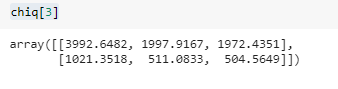Chi-Square Statistics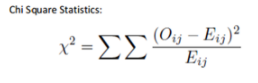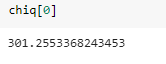## Correlation Analysis

Correlation analysis is used to measure the strength of the association (linear relationship) between two continuous variables

• Only concerned with the strength of the relationship
• No causal effect is implied
• The correlation coefficient ρ (rho) measures the strength of the association and also the direction of the relationship between two continuous variables
• Unit free
• The range between -1 and 1
• Close to -1, the stronger the negative linear relationship
• Close to 1, the stronger the positive linear relationship
• Close to 0, the weaker the linear relationship

Scenario:

In the data, we have Age and Estimated Salary, we want to check if these two variables have any linear relationship. We can use Pearson Correlation to do that.

`from scipy.stats.stats import pearsonr`

`pearsonr(attrition.Age, `

`         attrition.EstimatedSalary)`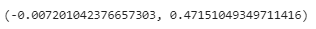Person Correlation is close to 0, it seems there is no relationship. The second parameter is P-Value can be used to assess the statistical significance of the association.

We can visualize the relationship using Scatter Plot.

`import matplotlib.pyplot as plt`

`plt.style.use('ggplot')`

`plt.xlabel('Age')`

`plt.ylabel('Estimated Salary')`

`plt.title ('Correlation Plot')`

`plt.scatter(attrition.Age, `

`         attrition.EstimatedSalary,`

`          marker="o")`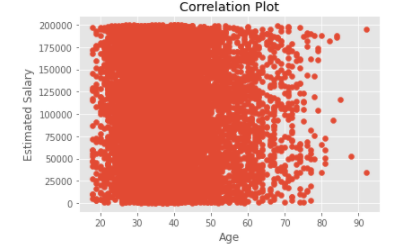TagsJul 17, 2021
Most PopularJun 18, 2020Jul 23, 2020Jun 19, 2020Jun 19, 2021